## Aspire's Library

A Place for Latest Exam wise Questions, Videos, Previous Year Papers,
Study Stuff for MCA Examinations

## NIMCET Previous Year Questions (PYQs)

#### NIMCET Progressions PYQ

NIMCET PYQ
The sum of infinite terms of a decreasing GP is equal to the greatest value of the function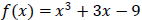in the interval [-2,3] and the difference between the first two terms is f'(0). Then the common ratio of GP is

NIMCET Previous Year PYQNIMCET NIMCET 2019 PYQ

#### Solution

NIMCET PYQ
If $a, b, c$ are in GP and $log a - log 2b$, $log 2b - log 3c$ and $log 3c - log a$ are in AP, then $a, b, c$are the lengths of the sides of a triangle which is

NIMCET Previous Year PYQNIMCET NIMCET 2019 PYQ

#### Solution

NIMCET PYQ

Not Available

NIMCET Previous Year PYQNIMCET NIMCET 2019 PYQ

#### Solution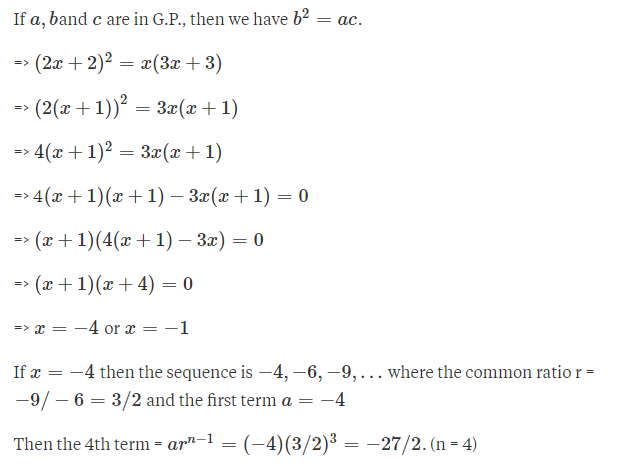NIMCET PYQ
If $a, a, a_2, ., a_{2n-1},b$ are in AP, $a, b_1, b_2,...b_{2n-1}, b$are in GP and $a, c_1, c_2,... c_{2n-1}, b$ are in HP, where a, b are positive, then the equation $a_n x^2-b_n+c_n$ has its roots

NIMCET Previous Year PYQNIMCET NIMCET 2019 PYQ

#### Solution

NIMCET PYQ
Which term of the series $\frac{\sqrt[]{5}}{3},\, \frac{\sqrt[]{5}}{4},\frac{1}{\sqrt[]{5}},\, ...$ is $\frac{\sqrt{5}}{13}$ ?

NIMCET Previous Year PYQNIMCET NIMCET 2022 PYQ

#### Solution

NIMCET PYQ
In a Harmonic Progression, $p^{th}$ term is $q$ and the $q^{th}$ term is $p$. Then $pq^{th}$ term is

NIMCET Previous Year PYQNIMCET NIMCET 2022 PYQ

#### Solution

NIMCET PYQ
Sum to infinity of a geometric is twice the sum of the first two terms. Then what are the possible values of common ratio?

NIMCET Previous Year PYQNIMCET NIMCET 2018 PYQ

#### Solution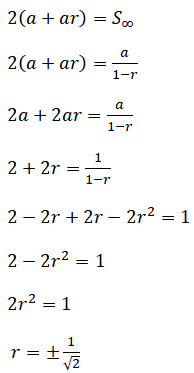NIMCET PYQ
Suppose that m and n are fixed numbers such that the mth  term am is equal to n and nth term an is equal to m, (m≠n), the the value of (m+n)th term  is

NIMCET Previous Year PYQNIMCET NIMCET 2018 PYQ

#### Solution

NIMCET PYQ
The harmonic mean of two numbers is 4. Their arithmetic mean A and the geometric mean G satisfy the relation 2A+G2 = 27, then the two numbers are

NIMCET Previous Year PYQNIMCET NIMCET 2017 PYQ

#### Solution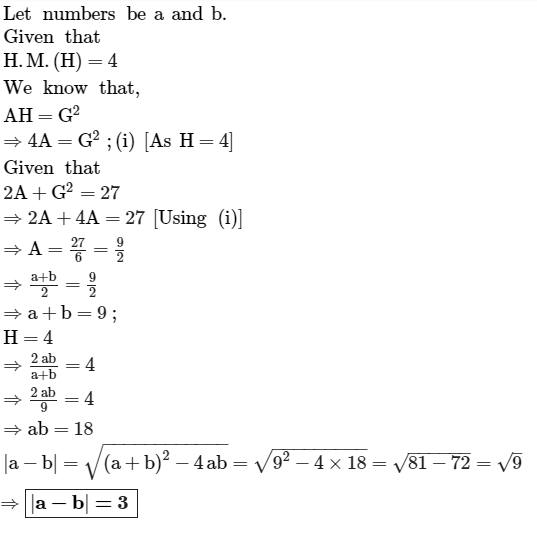NIMCET PYQ
Three positive number whose sum is 21 are in arithmetic progression. If 2, 2, 14 are added to them respectively then resulting numbers are in geometric progression. Then which of the following is not among the three numbers?

NIMCET Previous Year PYQNIMCET NIMCET 2017 PYQ

#### Solution

Let the three terms in A.P. be a – d, a, a + d.
given that a – d + a + a + d = 21
a = 7
then the three term in A.P. are 7 – d, 7, 7 + d
According to given condition 9 – d, 9, 21 + d are in G.P.
(9)2 = (9 – d) (21 + d)
81 = 189 + 9d – 21d – d2
81 = 189 – 12d – d2
d2 + 12d – 108 = 0
d(d + 18) – 6 (d + 18) = 0
(d – 6) (d + 18) = 0
We get, d = 6, –18
Putting d = 6 in the term 7 – d, 7, 7 + d we get 1, 7, 13.

NIMCET PYQ
If $H_1,H_2,\ldots,H_n$ are n harmonic means between a and b $(b\ne a)$;,then $\frac{{{H}}_n+a}{{{H}}_n-a}+\frac{{{H}}_n+b}{{{H}}_n-b}$

NIMCET Previous Year PYQNIMCET NIMCET 2021 PYQ

#### Solution

NIMCET PYQ
If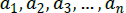are positive real numbers whose product is a fixed number c, then the minimum of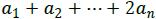is

NIMCET Previous Year PYQNIMCET NIMCET 2018 PYQ

#### Solution

NIMCET PYQ
The four geometric means between 2 and 64 are

NIMCET Previous Year PYQNIMCET NIMCET 2021 PYQ

#### Solution

NIMCET PYQ
An arithmetic progression has 3 as its first term. Also, the sum of the first 8 terms is twice the sum of the first 5 terms. Then what is the common difference?

NIMCET Previous Year PYQNIMCET NIMCET 2020 PYQ

#### Solution

NIMCET PYQ
The sum of infinite terms of decreasing GP is equal to the greatest value of the function $f(x) = x^3 + 3x – 9$ in the interval [–2, 3] and difference between the first two terms is f '(0). Then the common ratio of the GP is

NIMCET Previous Year PYQNIMCET NIMCET 2023 PYQ

#### Solution

NIMCET PYQ
If a, b, c, d are in HP and arithmetic mean of ab, bc, cd is 9 then which of the following number is the value ofad?

NIMCET Previous Year PYQNIMCET NIMCET 2023 PYQ

#### NIMCET

Online Test Series,
and More.

#### NIMCET

Online Test Series,